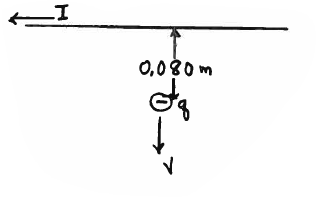# Problem: A long straight wire carries a current of I = 3.00 A directed to the left, as shown in the sketch. A small sphere with negative charge q = -5.00 x 10-4 C is moving from the wire. When the sphere is 0.080 m from the wire, its speed is v = 9.00 m/s. What are the magnitude and direction of the magnetic field produced by the wire at the location of the sphere, 0.080 m below the wire? Write the direction in the blank provided and also show the direction on the sketch. Ans. B = direction =

###### FREE Expert Solution
99% (473 ratings)
###### Problem Details

A long straight wire carries a current of I = 3.00 A directed to the left, as shown in the sketch. A small sphere with negative charge q = -5.00 x 10-4 C is moving from the wire. When the sphere is 0.080 m from the wire, its speed is v = 9.00 m/s. What are the magnitude and direction of the magnetic field produced by the wire at the location of the sphere, 0.080 m below the wire? Write the direction in the blank provided and also show the direction on the sketch.

Ans. B =

direction =